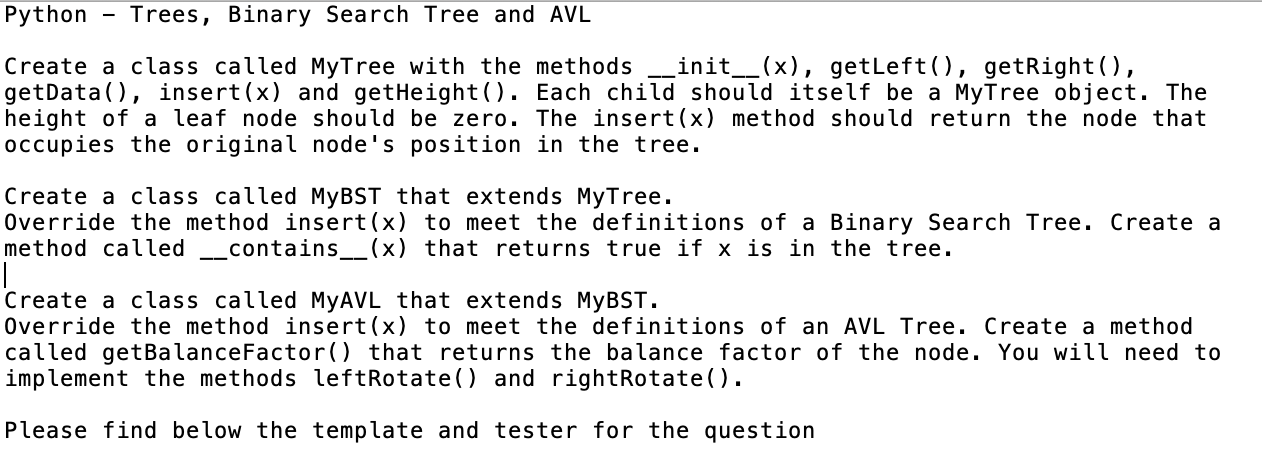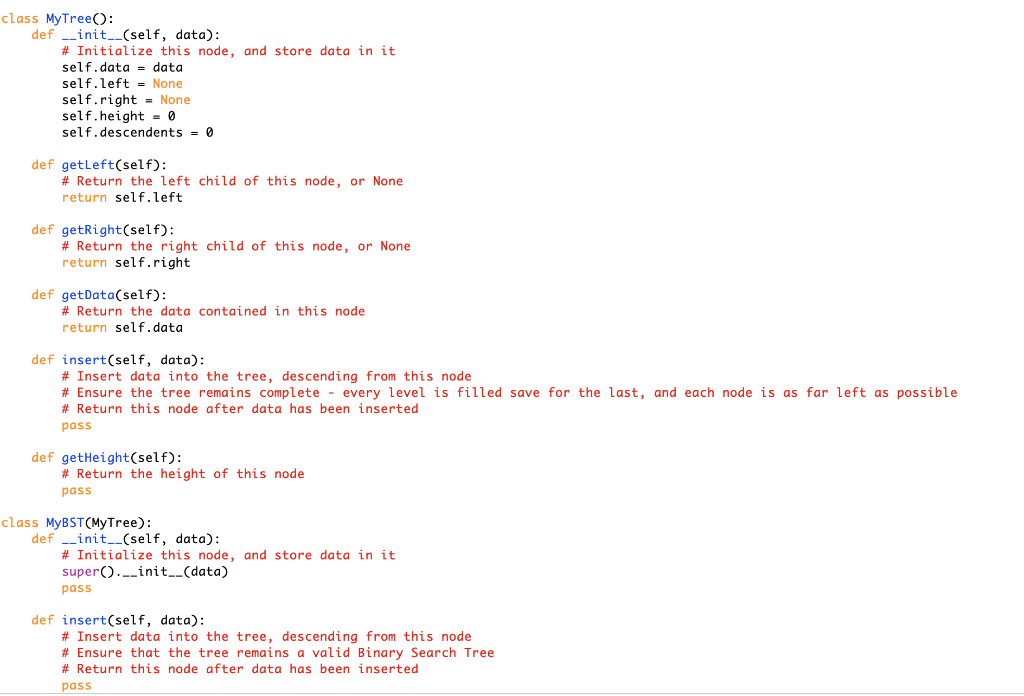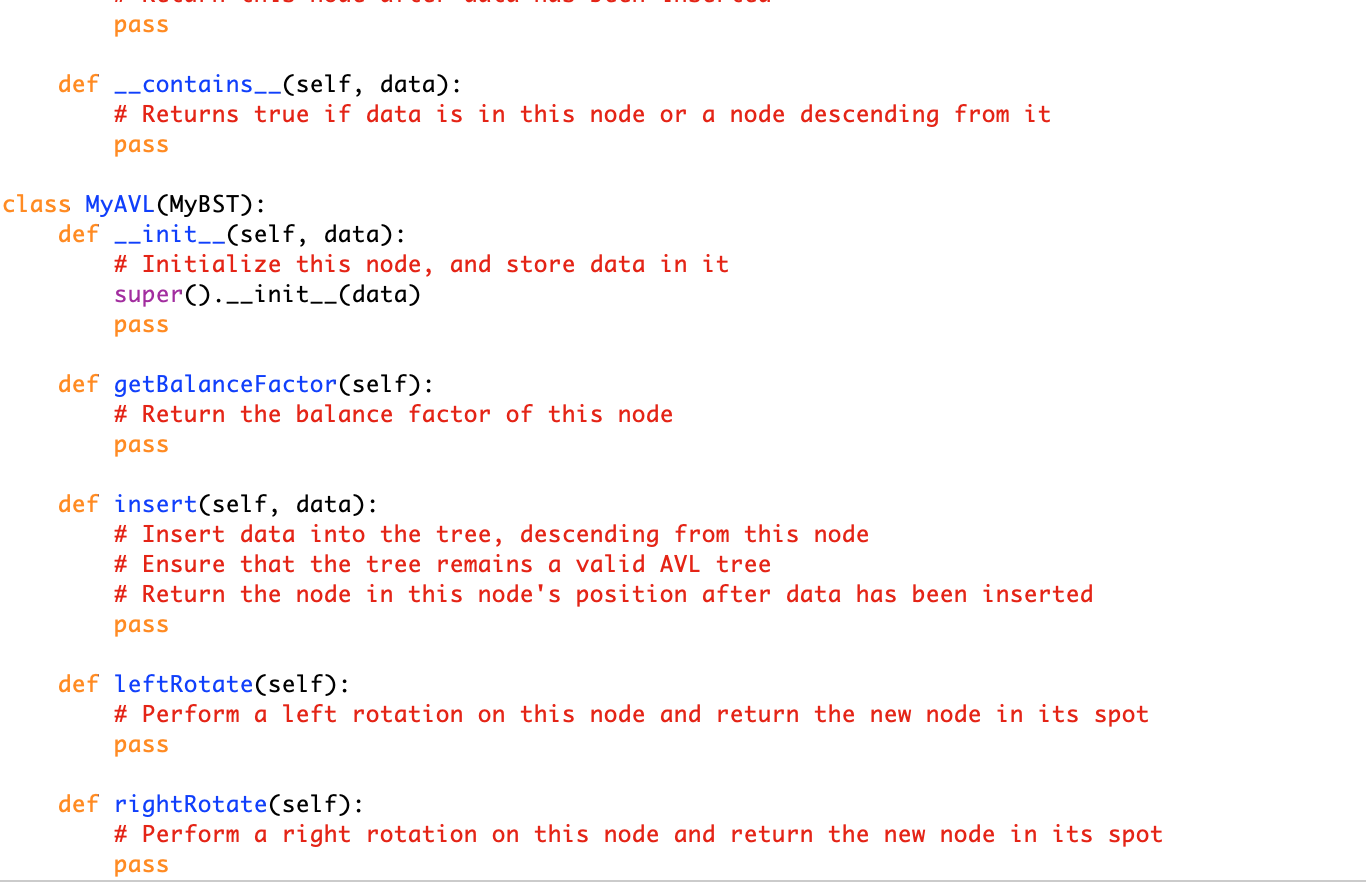# (Solved) : Templatepy Two Screen Shots One Script Avltesterpy Tester Import Random Module Imports Imp Q42769452 . . .#template.py (the two screen shots are in one script)#avltester.py (tester) import random#Module Importsimport sysfrom importlib import import_module def CheckHeight(tree):    if tree is None:        return -1    else:        returnmax(CheckHeight(tree.getLeft())+1,CheckHeight(tree.getRight())+1) def CheckAVL(tree):    if CheckHeight(tree) > 0:        l = 0 if tree.getLeft()is None else CheckHeight(tree.getLeft())+1        r = 0 if tree.getRight()is None else CheckHeight(tree.getRight())+1        b = l-r        if abs(b) >= 2 or b!= tree.getBalanceFactor():           print(f”balance factor is {b} and tree claims it is{tree.getBalanceFactor()}”)           printTree(tree)           return False        else:           return CheckAVL(tree.getLeft()) and CheckAVL(tree.getRight())    else:        return True def printTree_(tree, prefix):    print(f”{prefix}{tree.data}”)    if tree.getLeft() is not None:       printTree_(tree.getLeft(),prefix+”-“)    if tree.getRight() is not None:       printTree_(tree.getRight(),prefix+”-“) def printTree(tree):    printTree_(tree,””) def Test(lib, seed=0, size=10, rounds=10, verbose=False):    random.seed(a=seed)     # Test MyTree     n = random.randint(0, size)    try:        tree =lib.MyTree(n)    except:        if verbose:           print(“Error: MyTree not creatable”)        return False       h=1    c=2    for i in range(1,size):        n =random.randint(n,n*2)        try:           tree = tree.insert(n)        except:           print(c)           if verbose:               print(“Error: Tree not insertable”)           return False         tH =tree.getHeight()        if CheckHeight(tree) !=tH:           if verbose:               print(“Error: Tree height calculation incorrect”)           return False         if tH != h:           if verbose:               print(“Error: Tree height incorrect: Should be {h} but is{tH}”)           return False                  if i == c:           h+=1           c+=(2**h)     del(tree)    if verbose:        print(“Tree testcomplete.”)    # Tree works    yield True     m = size*3    n = random.randint(size, size*2)     try:        bst = lib.MyBST(n)    except:        if verbose:           print(“Error: MyBST not creatable”)        return False     try:       bst=bst.insert(n+1)    except:        if verbose:           print(“Error: BST not insertable”)        return False     try:       bst=bst.insert(n-1)    except:        if verbose:           print(“Error: BST not insertable”)        return False     if bst.getHeight() != 1 or bst.getHeight() !=CheckHeight(bst):        if verbose:           print(“Error: BST height incorrect”)        return False      try:       bst=bst.insert(n+2)       bst=bst.insert(n+3)    except:        if verbose:           print(“Error: BST not insertable”)        return False     if bst.getHeight() != 3 or bst.getHeight() !=CheckHeight(bst):        if verbose:           print(“Error: BST height incorrect.”)        return False         del(bst)    bst= lib.MyBST(n)     a = []    for i in range(size):        v =random.randint(0,m)        bst= bst.insert(v)        if not(v in a):           a.append(v)          for i in range(size):        if len(a) >=size:           m*=2        v =random.randint(0,m)        if (v in a) != (v inbst):           if verbose:               print(“Error: BST Search incorrect”)           return False       del(bst)     if verbose:        print(“BST testcomplete.”)      yield True #BST works     n=10    try:        avl = lib.MyAVL(n)    except:        if verbose:           print(“Error: MyAVL not creatable”)        return False    first = avl    try:        avl =avl.insert(n+6)    except:        if verbose:           print(“Error: AVL not insertable #1”)        return False    try:        avl =avl.insert(n+12)    except:        if verbose:           print(“Error: AVL not insertable #2”)        return False     if not(first is avl.getLeft()):        if verbose:           print(“Error: AVL Rotation incorrect #1”)        return False     try:        second =avl.getRight()        second    except:        if verbose:           print(“Error: AVL Node lost during rotation”)        return False     try:        avl=avl.insert(8)        avl=avl.insert(6)    except:        if verbose:           print(“Error: AVL Node not insertable”)        return False     if not(first isavl.getLeft().getRight()):        if verbose:           print(“Error: AVL Rotation incorrect #2”)        return False      if verbose:        print(“AVL DoubleRotation test complete”)      yield True # Simple rotation correct     try:       avl=avl.insert(n-3)    except:        if verbose:           print(“Error: AVL Node not insertable”)        return False     # Force rotations    avl = avl.insert(n-2)    avl = avl.insert(n-1)    avl = avl.insert(n*2+4)    avl = avl.insert(n*2+3)     if not (first isavl.getRight().getLeft().getRight()):        if verbose:           print(“Error: AVL Rotation incorrect #3”)        return False      if verbose:        print(“AVL DoubleRotation test complete”)    yield True # Rotation test complete     for i in range(0, size):        avl =avl.insert(random.randint(0,size))     if not CheckAVL(avl):        if verbose:           print(“Error: AVL Property not maintained across inserts”)        return False     if verbose:        print(“AVL Property testcomplete”)     yield True # Big test complete if __name__ == “__main__”:    if len(sys.argv) < 2:        print(“Include at leasta library name as an argument.”)        exit()    name = sys.argv    if name.endswith(“.py”):        name = name[:-3]    print(f”Testing module {name}”)   module=import_module(name,package=__name__)    score=0    for i in Test(module,seed=123456, size=1000,rounds=200, verbose=True):        if i:           score+=1        else:           break     print(f”Test result: {score}/5″)

Python Trees, Binary Search Tree and AVL init__(x), getLeft(), getRight (), Create a class called MyTree with the methods getData (), insert(x) and getHeight(). Each child should itself be a MyTree object. The height of a leaf node should be zero. The insert(x) method should return the node that occupies the original node’s position in the tree. Create a class called MyBST that extends MyTree. Override the method insert(x) to meet the definitions of a Binary Search Tree. Create a method called _contains__(x) that returns true if x is in the tree. Create a class called MYAVL that extends MyBST. Override the method insert(x) to meet the definitions of an AVL Tree. Create a method called getBalanceFactor() that returns the balance factor of the node. You will need to implement the methods leftRotate() and rightRotate() Please find below the template and tester for the question class MyTree): definit_(self, data) Initialize this node, and store data in it self.data = data self.left None self.right =None self.height = 0 self.descendents = 0 def getLeft(sel f) # Return the left child of this node, or None return self.left def getRight(self): #Return the right child of this node, or None return self.right def getData(self) # Return the data contained in this node return self. data def insert(self, data): # Insert data into the tree, descending from this node # Ensure the tree remains complete every level is filled save for the last, and each node is as far left as possible # Return this node after data has been inserted pass def getHeight(self): # Return the height of this node pass class MyBST(MyTree): def init_(self, data) # Initialize this node, and store data in it super.init__(data) pass def insert(self, data) # Insert data into the tree, descending from this node # Ensure that the tree remains a valid Binary Search Tree # Return this node after data has been inserted pass pass def _contains__(self, data): #Returns true if data is in this node or a node descending from it pass class MyAVL(MYBST): def _init__(self, data): # Initialize this node, and store data in it super()._init__(data) pass def getBalanceFactor(self): #Return the balance factor of this node pass def insert(self, data): # Insert data into the tree, descending from this node # Ensure that the tree remains a valid AVL tree # Return the node in this node’s position after data has been inserted pass def leftRotate(self): #Perform a left rotation on this node and return the new node in its spot pass def rightRotate(self): # Perform a right rotation on this node and return the new node in its spot pass Show transcribed image text Python Trees, Binary Search Tree and AVL init__(x), getLeft(), getRight (), Create a class called MyTree with the methods getData (), insert(x) and getHeight(). Each child should itself be a MyTree object. The height of a leaf node should be zero. The insert(x) method should return the node that occupies the original node’s position in the tree. Create a class called MyBST that extends MyTree. Override the method insert(x) to meet the definitions of a Binary Search Tree. Create a method called _contains__(x) that returns true if x is in the tree. Create a class called MYAVL that extends MyBST. Override the method insert(x) to meet the definitions of an AVL Tree. Create a method called getBalanceFactor() that returns the balance factor of the node. You will need to implement the methods leftRotate() and rightRotate() Please find below the template and tester for the question
class MyTree): definit_(self, data) Initialize this node, and store data in it self.data = data self.left None self.right =None self.height = 0 self.descendents = 0 def getLeft(sel f) # Return the left child of this node, or None return self.left def getRight(self): #Return the right child of this node, or None return self.right def getData(self) # Return the data contained in this node return self. data def insert(self, data): # Insert data into the tree, descending from this node # Ensure the tree remains complete every level is filled save for the last, and each node is as far left as possible # Return this node after data has been inserted pass def getHeight(self): # Return the height of this node pass class MyBST(MyTree): def init_(self, data) # Initialize this node, and store data in it super.init__(data) pass def insert(self, data) # Insert data into the tree, descending from this node # Ensure that the tree remains a valid Binary Search Tree # Return this node after data has been inserted pass
pass def _contains__(self, data): #Returns true if data is in this node or a node descending from it pass class MyAVL(MYBST): def _init__(self, data): # Initialize this node, and store data in it super()._init__(data) pass def getBalanceFactor(self): #Return the balance factor of this node pass def insert(self, data): # Insert data into the tree, descending from this node # Ensure that the tree remains a valid AVL tree # Return the node in this node’s position after data has been inserted pass def leftRotate(self): #Perform a left rotation on this node and return the new node in its spot pass def rightRotate(self): # Perform a right rotation on this node and return the new node in its spot pass

Answer to #template.py (the two screen shots are in one script) #avltester.py (tester) import random #Module Imports import sys fr…

We are the best freelance writing portal. Looking for online writing, editing or proofreading jobs? We have plenty of writing assignments to handle.